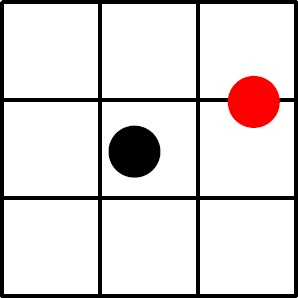# Win that stuffed toy

Probability Level 4

At the local games arcade, you have to toss a circular disc of diameter 5 onto a grid of $3 \times 3$ squares, each of which has side length 10. You win a stuffed toy if your disc does not touch any of the grid lines. You are playing the kiddie version, where walls are set up on the sides to ensure that the disc will be contained entirely within the grid. Assume that the position of your thrown disc is distributed uniformly, the probability that it lands entirely in a grid square can be expressed as $\frac{a}{b}$ where $a$ and $b$ are coprime positive integers. What is the value of $a + b$?

Details and assumptions

In the figure below, the black disc is entirely in a grid square, but the red disc is not.×